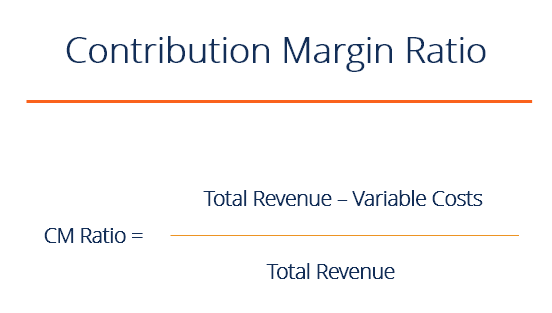# Contribution Margin Ratio

The formula to determine the contribution margin of a business.

## Contribution margin ratio formula

The contribution margin ratio (CM ratio) of a business is equal to its revenue less all variable costs – divided by its revenue. It represents the marginal benefit of producing one more unit.  Here is the formula for contribution margin ratio (CM ratio):See an example in Excel here.

### Example calculation of contribution margin ratio

CM ratio = (total revenue – cost of goods sold – any other variable expenses) / total revenue

A company has revenue of \$50 million, cost of good sold is \$20 million, marketing is \$5 million, product delivery fees are \$5 million and fixed costs are \$10 million.

Contribution margin dollars = \$50M – \$20M – \$5M – \$5M = \$20 million

Contribution margin ratio = \$20M / \$50M = 40%

The fixed costs of \$10 million are not included in the formula, however, it is important to make sure the CM dollars are greater than the fixed costs, otherwise, the company is not profitable.

### Breakeven analysis

Contribution margin is not necessarily a good indication of economic benefit.  Companies may have significant fixed costs that need to be factored in.

It can be important to perform a breakeven analysis to determine how many units need to be sold, and at what price, in order for a company to break even.  To learn more, check out our Financial Analysis course.

In order to perform this analysis, calculate the contribution margin per unit, then divide the fixed costs by this number and you will know how many units you have to sell to break even.

Building on the above example, suppose that company sold 1 million units.  That means the CM per unit is \$20.  Fixed costs are \$10 million so the company has to sell 500,000 units to break even. (\$10 million / \$20 per unit = 500,000).

### More learning

• Profit Margin
• Leverage Ratios
• What is Financial Modeling
• Financial Modeling for Dummies
• How to be a great financial analyst

To find out more about finance careers, try out our interactive Career Map.

### Financial Analyst Training

Get world-class financial training with CFI’s online certified financial analyst training program!

Gain the confidence you need to move up the ladder in a high powered corporate finance career path.

Learn financial modeling and valuation in Excel the easy way, with step-by-step training.